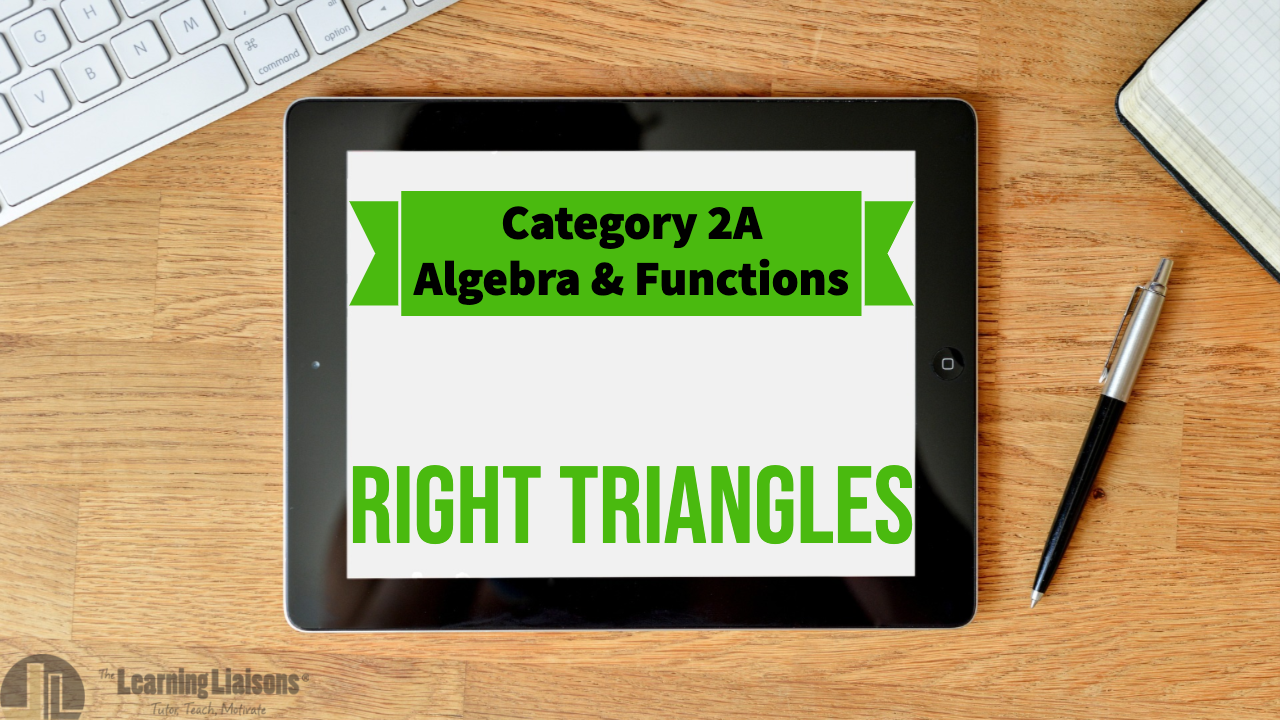# Update 7: Right Triangles

Praxis Core Mathematics (5732): Geometry
Skill: 3B Right Triangles

Praxis Core Mathematics (5733): not tested

Problems included:
- Solving for a missing side of a right triangle
- Word Problems involving the Pythagorean Theorem
- Solving for side lengths using the Pythagorean Theorem